Скачать презентацию AI Representation models and Propositional Logic Why do

274f360a36bafa323ea843cf47987322.ppt

• Количество слайдов: 51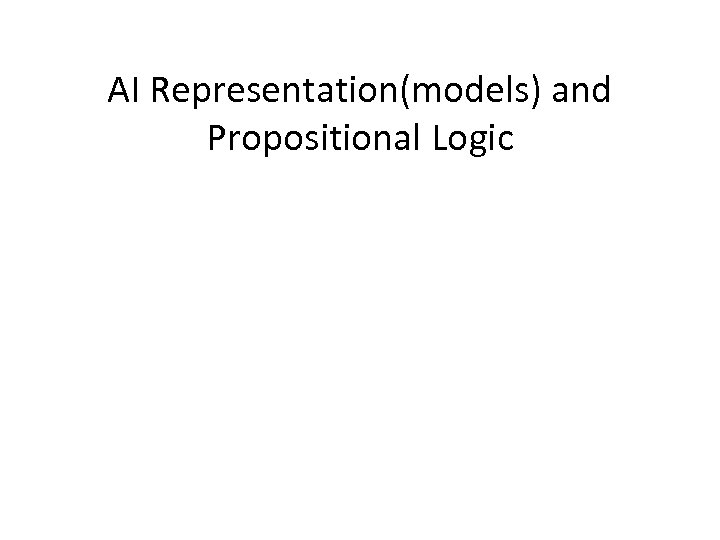AI Representation(models) and Propositional Logic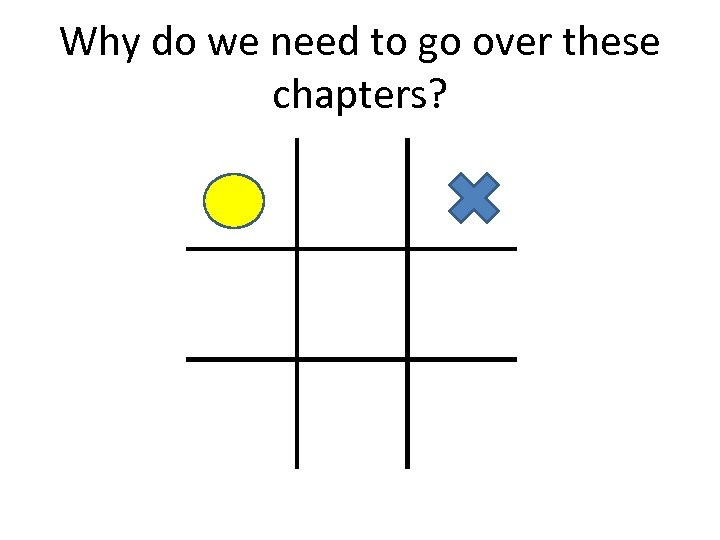Why do we need to go over these chapters?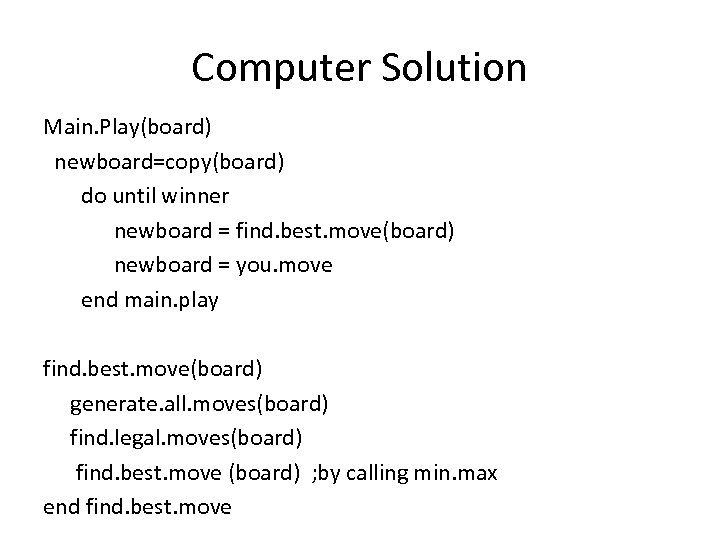Computer Solution Main. Play(board) newboard=copy(board) do until winner newboard = find. best. move(board) newboard = you. move end main. play find. best. move(board) generate. all. moves(board) find. legal. moves(board) find. best. move (board) ; by calling min. max end find. best. move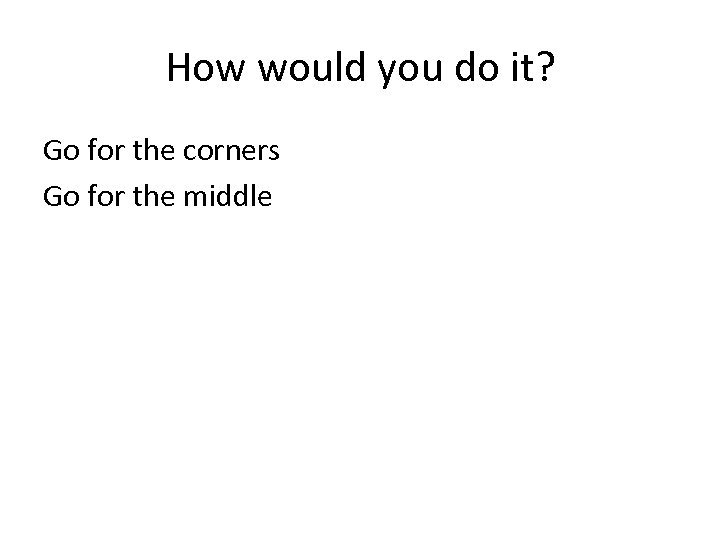How would you do it? Go for the corners Go for the middle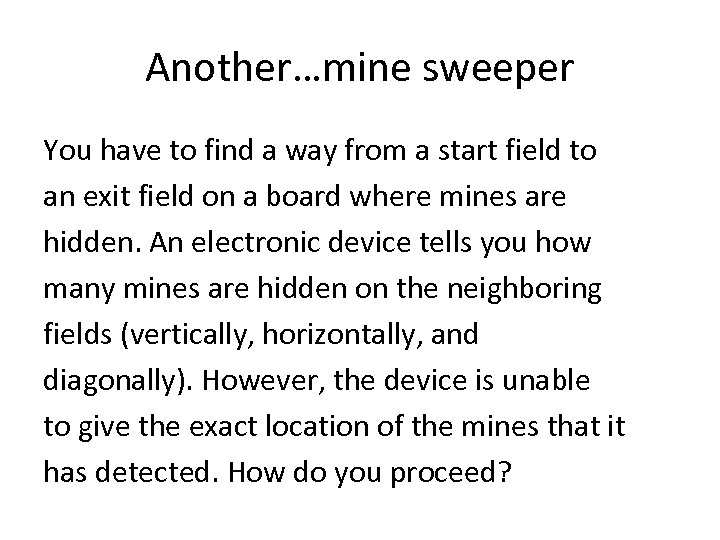Another…mine sweeper You have to find a way from a start field to an exit field on a board where mines are hidden. An electronic device tells you how many mines are hidden on the neighboring fields (vertically, horizontally, and diagonally). However, the device is unable to give the exact location of the mines that it has detected. How do you proceed?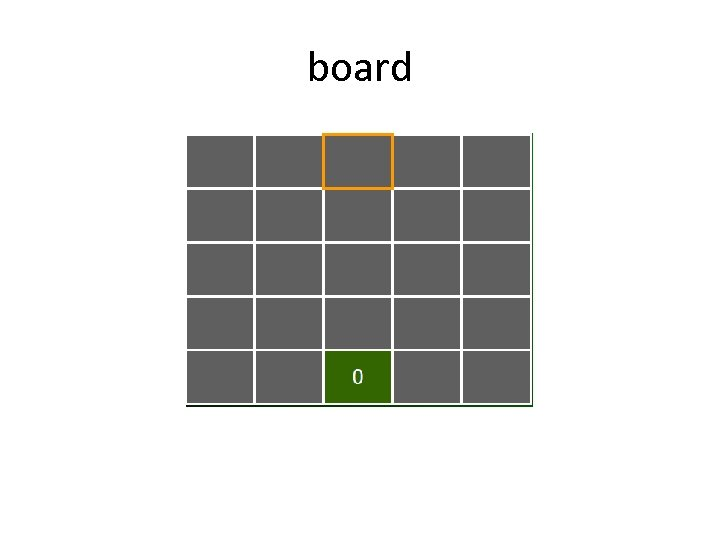board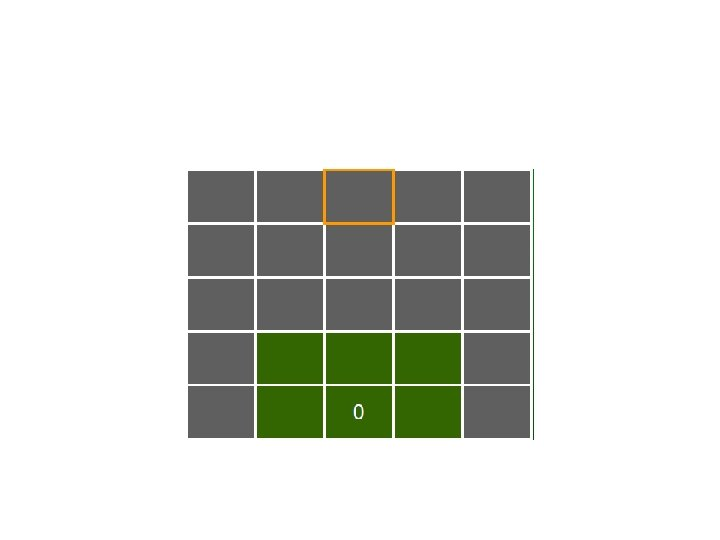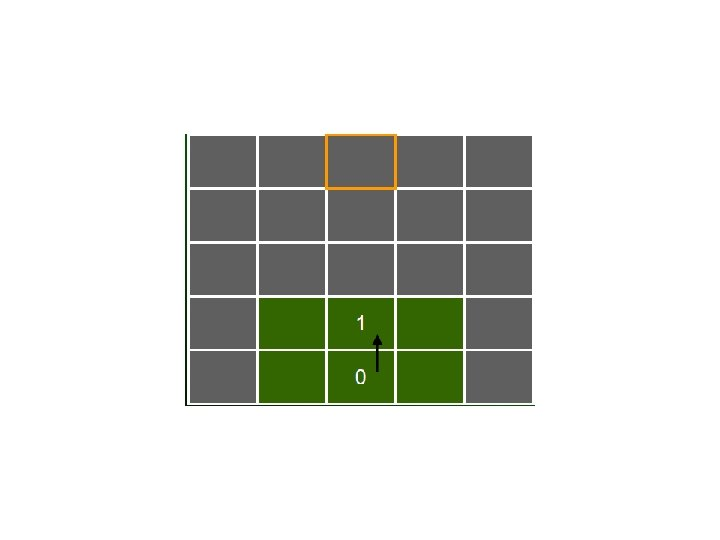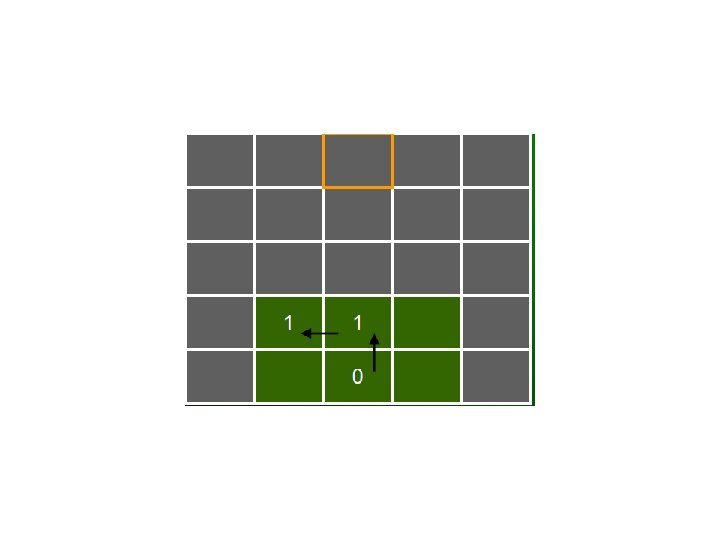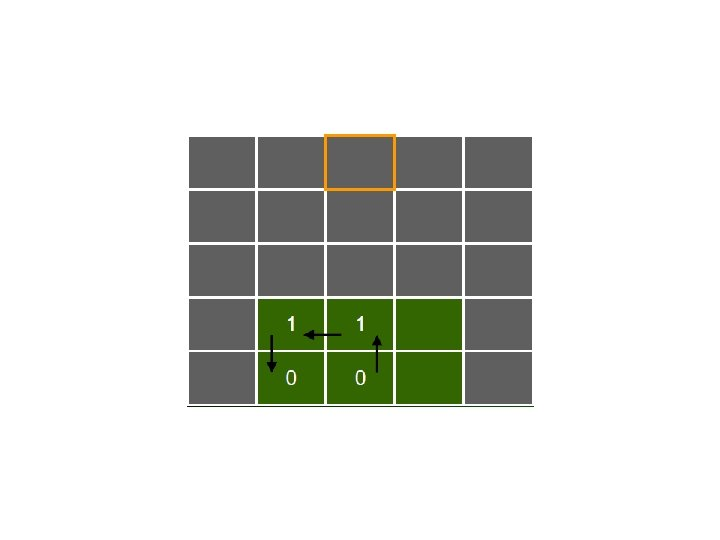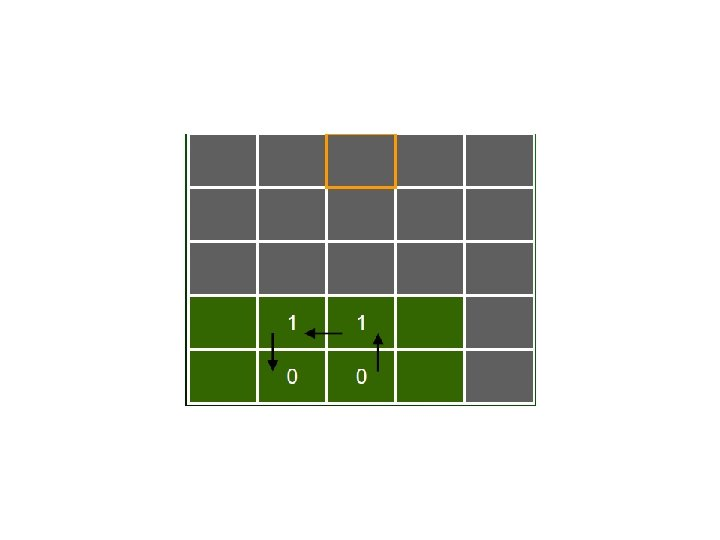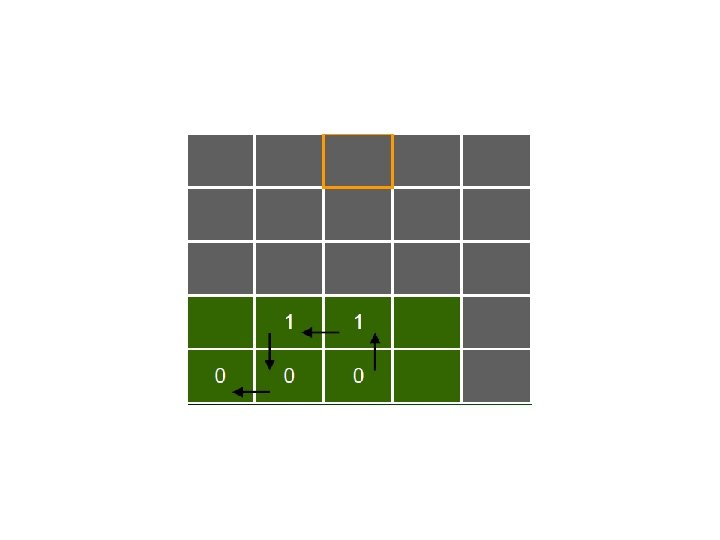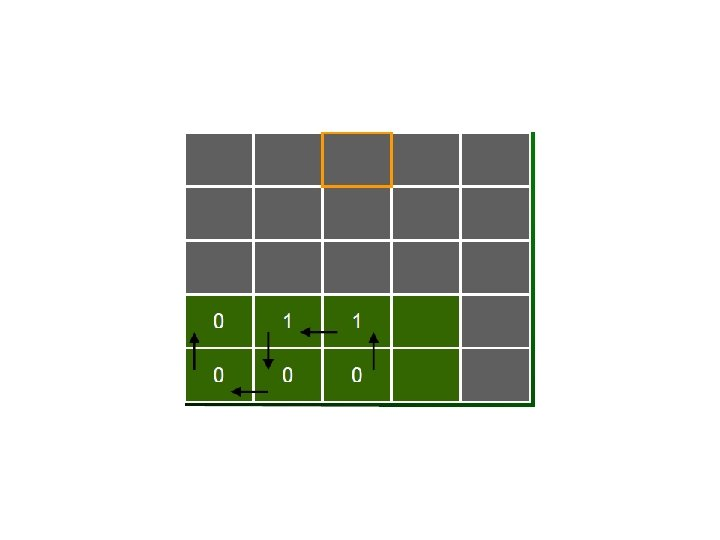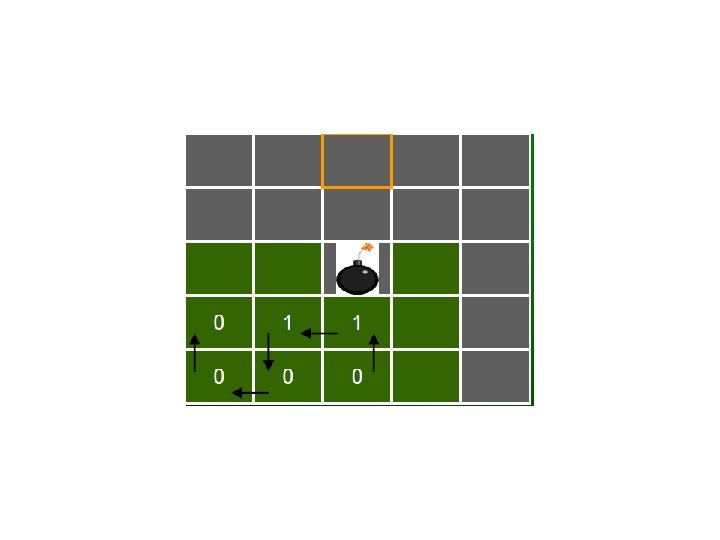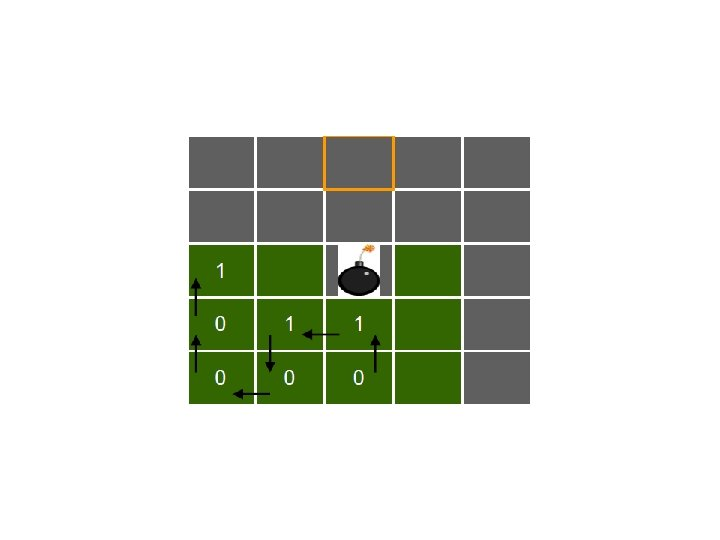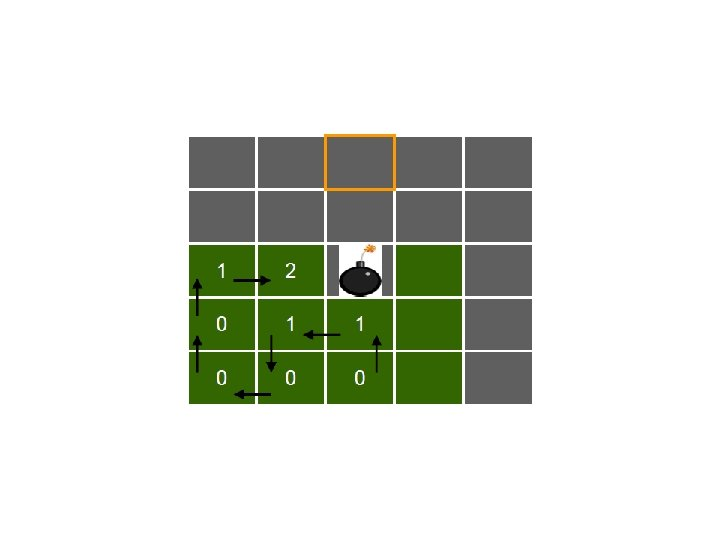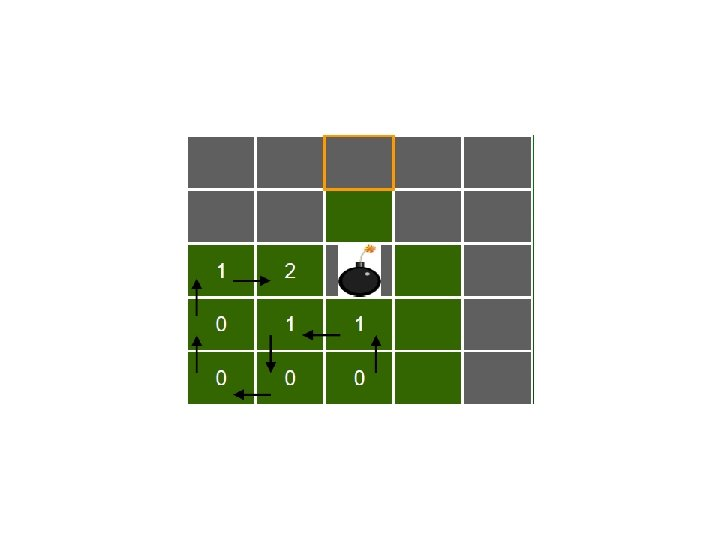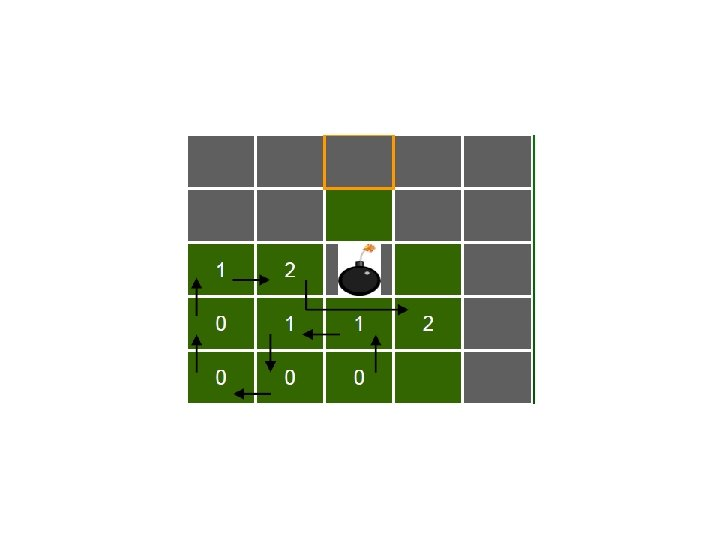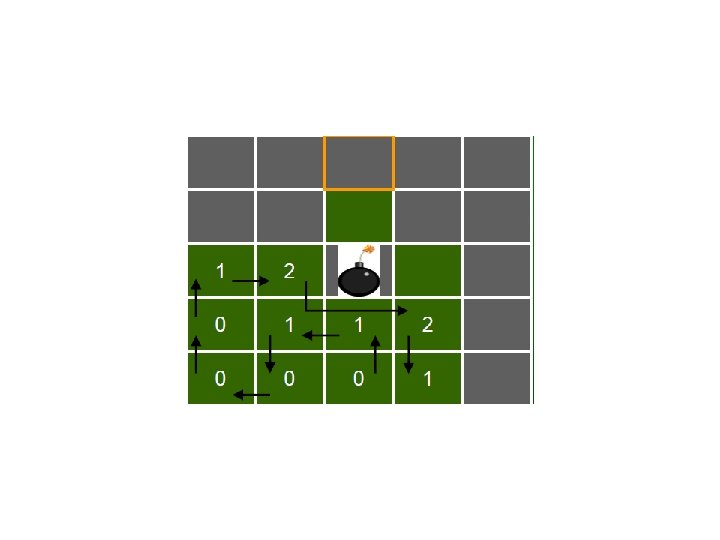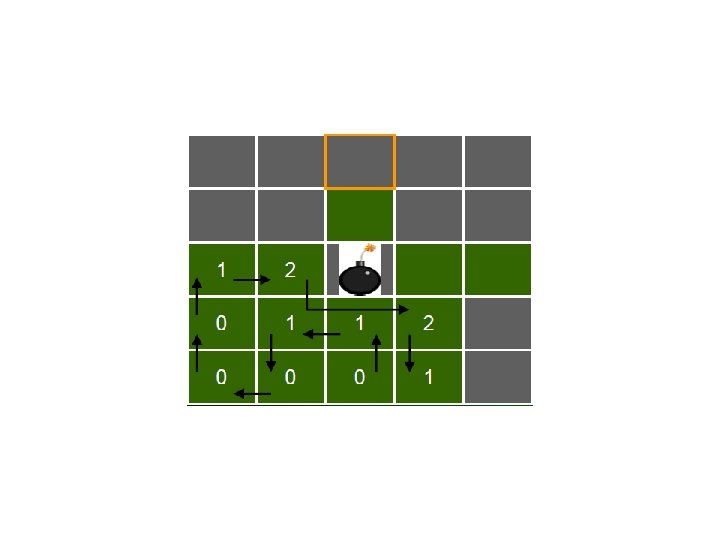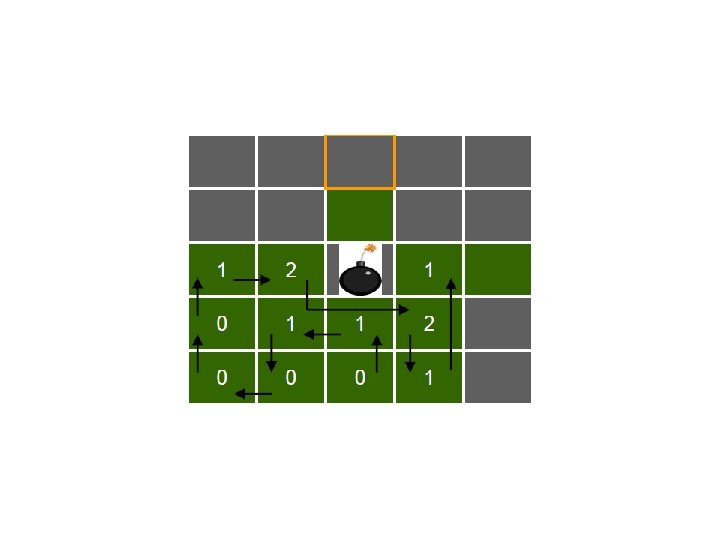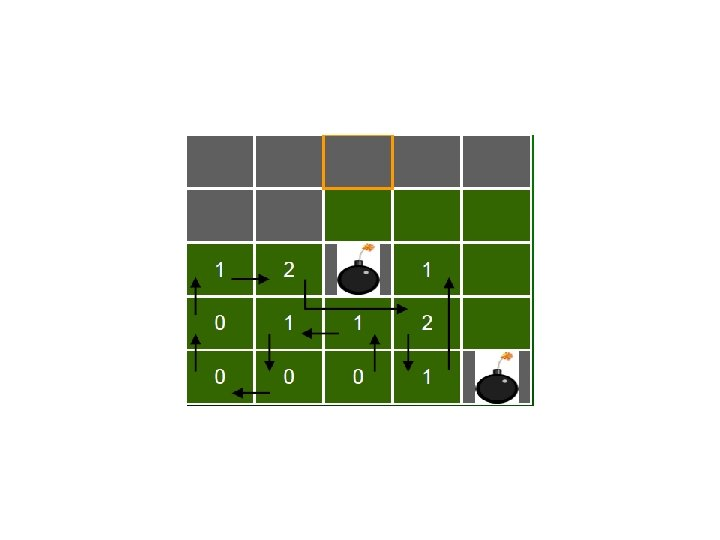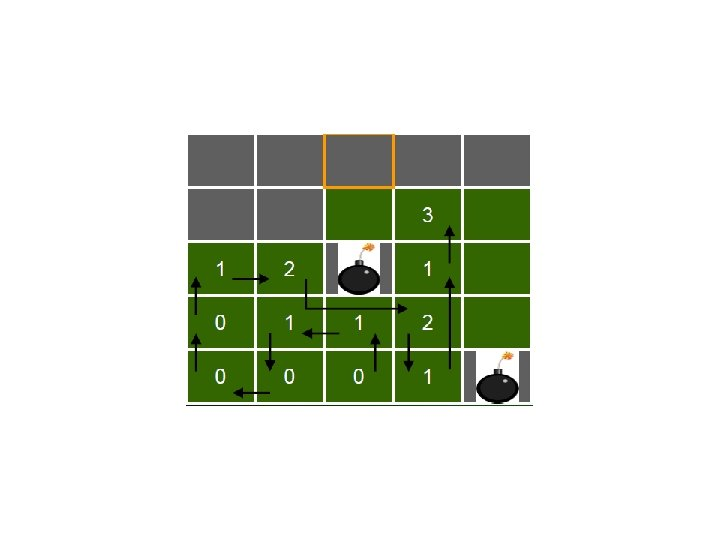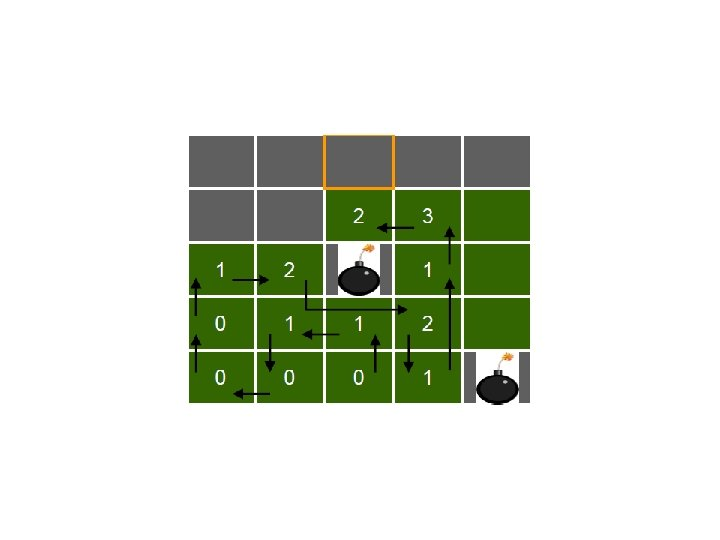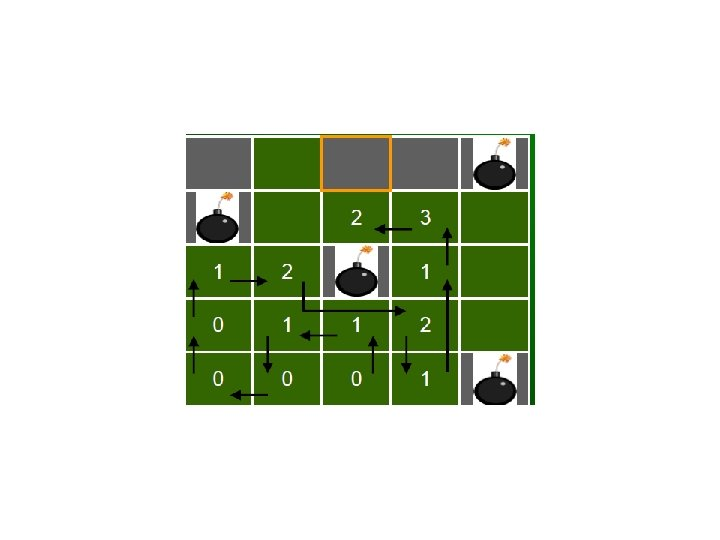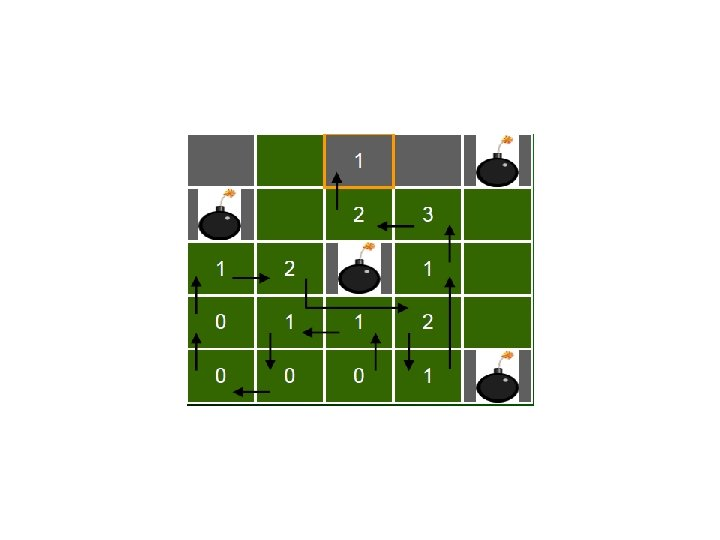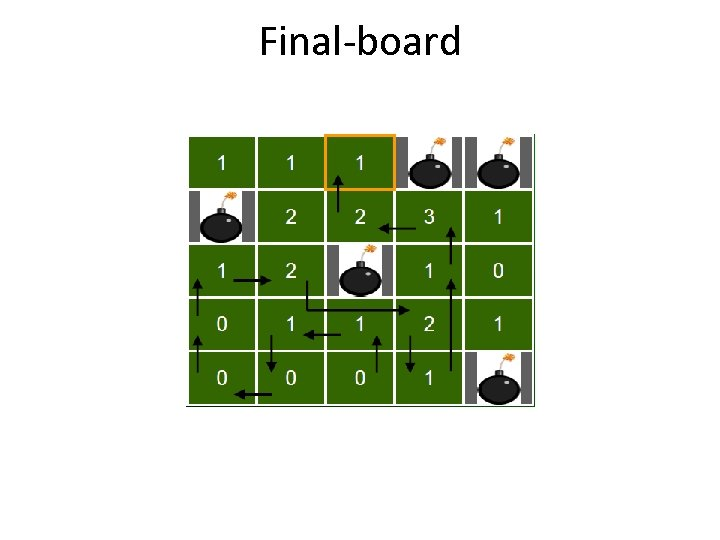Final-board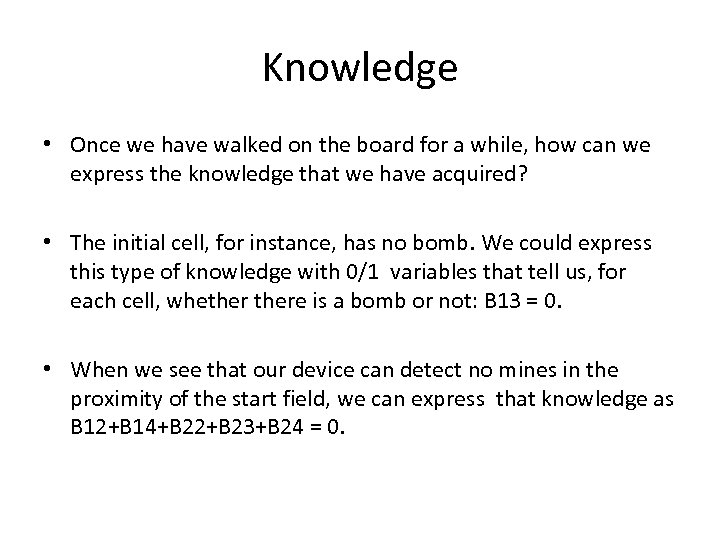Knowledge • Once we have walked on the board for a while, how can we express the knowledge that we have acquired? • The initial cell, for instance, has no bomb. We could express this type of knowledge with 0/1 variables that tell us, for each cell, whethere is a bomb or not: B 13 = 0. • When we see that our device can detect no mines in the proximity of the start field, we can express that knowledge as B 12+B 14+B 22+B 23+B 24 = 0.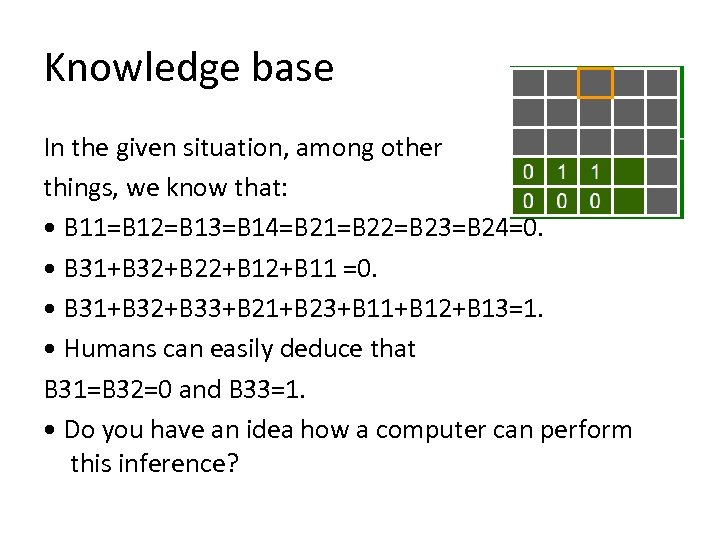Knowledge base In the given situation, among other things, we know that: • B 11=B 12=B 13=B 14=B 21=B 22=B 23=B 24=0. • B 31+B 32+B 22+B 11 =0. • B 31+B 32+B 33+B 21+B 23+B 11+B 12+B 13=1. • Humans can easily deduce that B 31=B 32=0 and B 33=1. • Do you have an idea how a computer can perform this inference?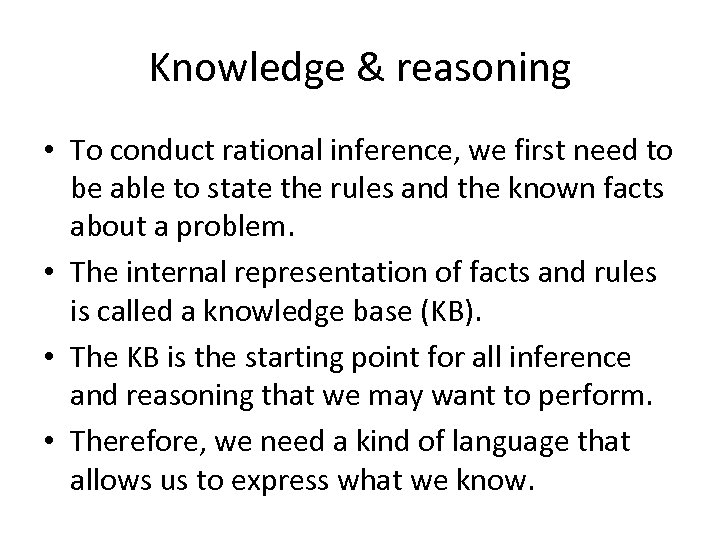Knowledge & reasoning • To conduct rational inference, we first need to be able to state the rules and the known facts about a problem. • The internal representation of facts and rules is called a knowledge base (KB). • The KB is the starting point for all inference and reasoning that we may want to perform. • Therefore, we need a kind of language that allows us to express what we know.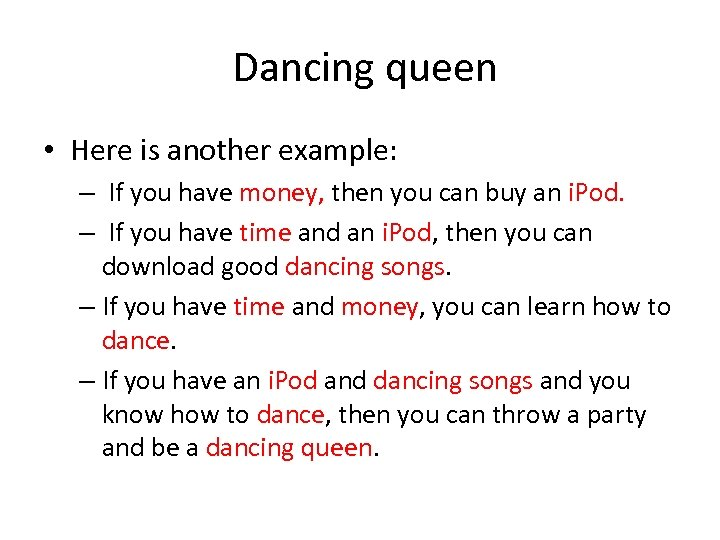Dancing queen • Here is another example: – If you have money, then you can buy an i. Pod. – If you have time and an i. Pod, then you can download good dancing songs. – If you have time and money, you can learn how to dance. – If you have an i. Pod and dancing songs and you know how to dance, then you can throw a party and be a dancing queen.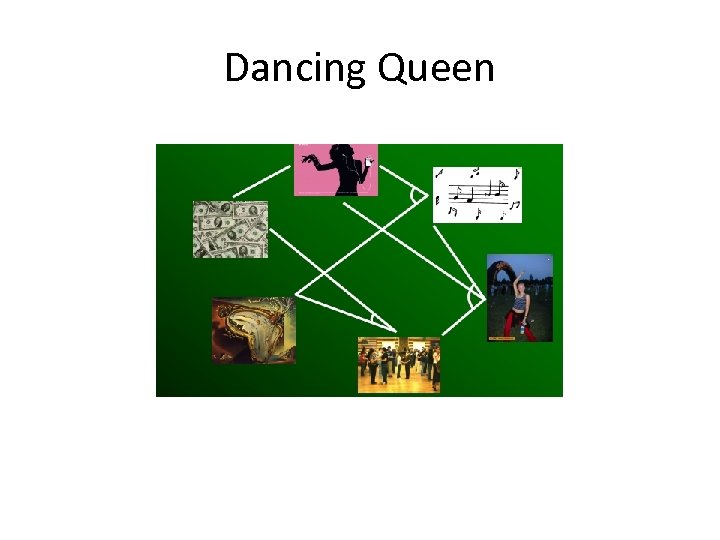Dancing Queen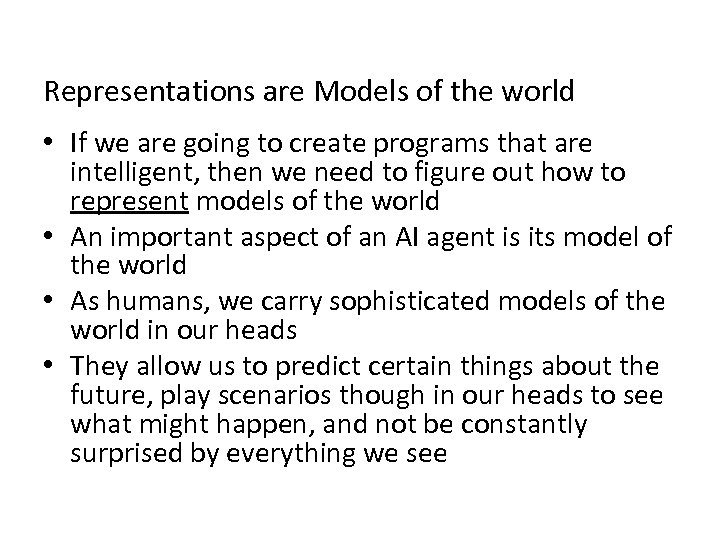Representations are Models of the world • If we are going to create programs that are intelligent, then we need to figure out how to represent models of the world • An important aspect of an AI agent is its model of the world • As humans, we carry sophisticated models of the world in our heads • They allow us to predict certain things about the future, play scenarios though in our heads to see what might happen, and not be constantly surprised by everything we see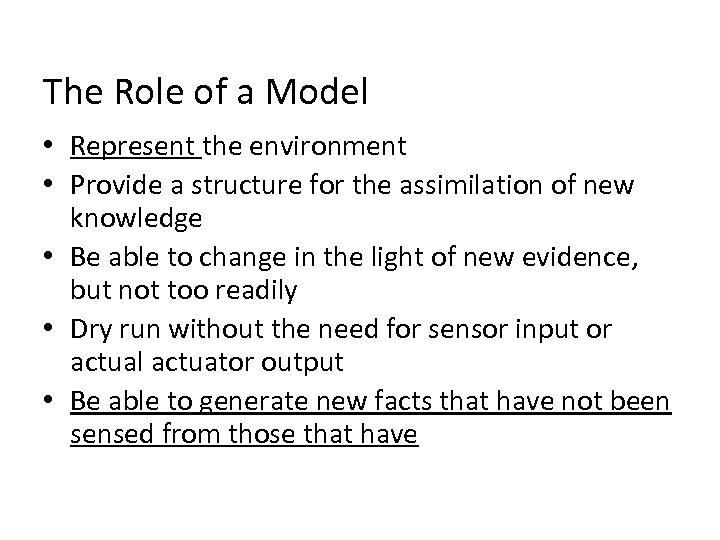The Role of a Model • Represent the environment • Provide a structure for the assimilation of new knowledge • Be able to change in the light of new evidence, but not too readily • Dry run without the need for sensor input or actual actuator output • Be able to generate new facts that have not been sensed from those that have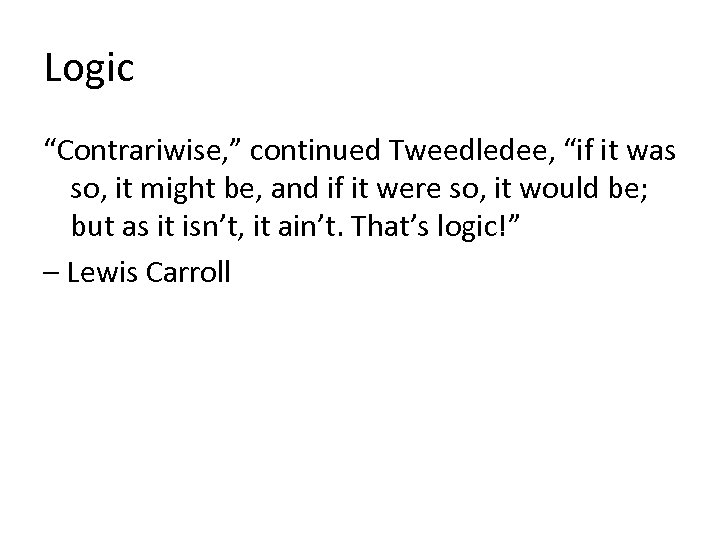Logic “Contrariwise, ” continued Tweedledee, “if it was so, it might be, and if it were so, it would be; but as it isn’t, it ain’t. That’s logic!” – Lewis Carroll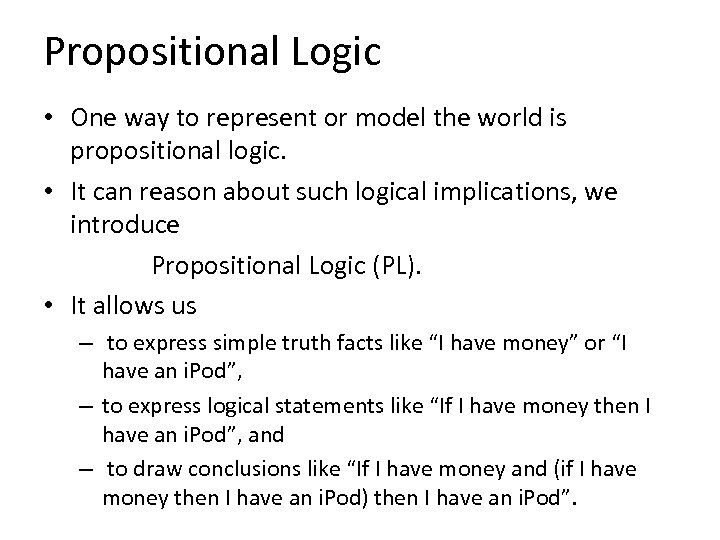Propositional Logic • One way to represent or model the world is propositional logic. • It can reason about such logical implications, we introduce Propositional Logic (PL). • It allows us – to express simple truth facts like “I have money” or “I have an i. Pod”, – to express logical statements like “If I have money then I have an i. Pod”, and – to draw conclusions like “If I have money and (if I have money then I have an i. Pod) then I have an i. Pod”.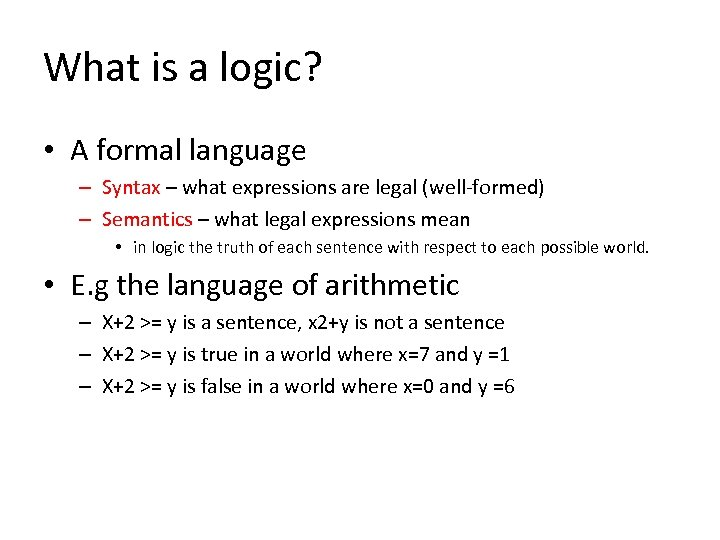What is a logic? • A formal language – Syntax – what expressions are legal (well-formed) – Semantics – what legal expressions mean • in logic the truth of each sentence with respect to each possible world. • E. g the language of arithmetic – X+2 >= y is a sentence, x 2+y is not a sentence – X+2 >= y is true in a world where x=7 and y =1 – X+2 >= y is false in a world where x=0 and y =6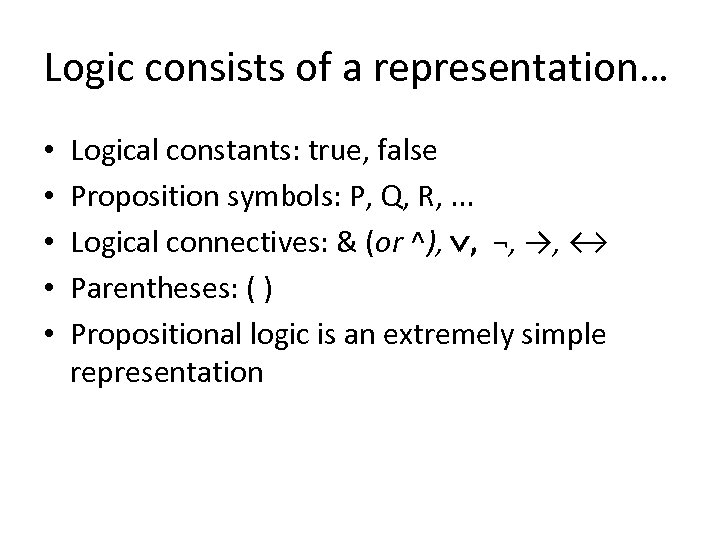Logic consists of a representation… • • • Logical constants: true, false Proposition symbols: P, Q, R, . . . Logical connectives: & (or ^), , ¬, →, ↔ Parentheses: ( ) Propositional logic is an extremely simple representation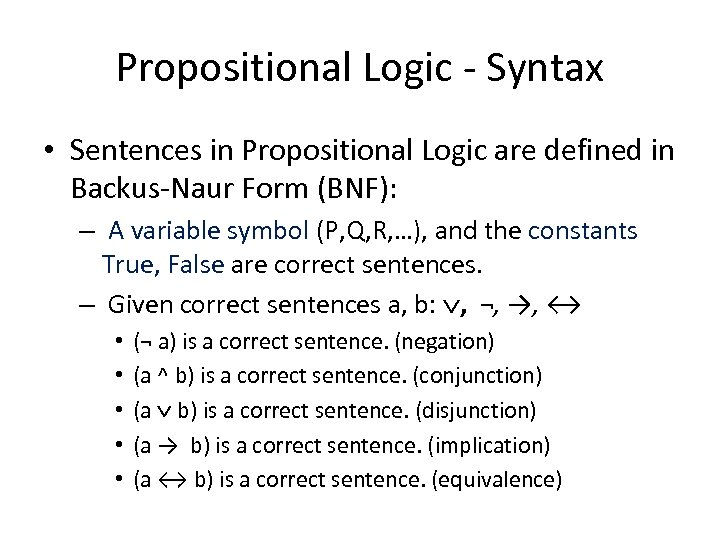Propositional Logic - Syntax • Sentences in Propositional Logic are defined in Backus-Naur Form (BNF): – A variable symbol (P, Q, R, …), and the constants True, False are correct sentences. – Given correct sentences a, b: , ¬, →, ↔ • • • (¬ a) is a correct sentence. (negation) (a ^ b) is a correct sentence. (conjunction) (a b) is a correct sentence. (disjunction) (a → b) is a correct sentence. (implication) (a ↔ b) is a correct sentence. (equivalence)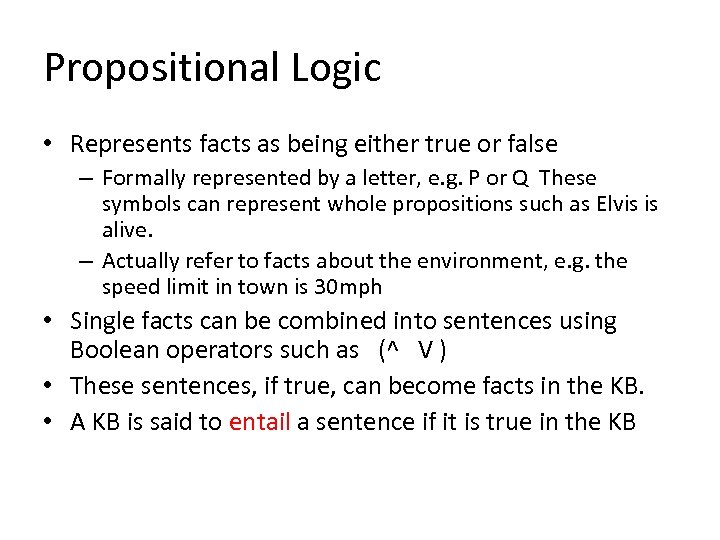Propositional Logic • Represents facts as being either true or false – Formally represented by a letter, e. g. P or Q These symbols can represent whole propositions such as Elvis is alive. – Actually refer to facts about the environment, e. g. the speed limit in town is 30 mph • Single facts can be combined into sentences using Boolean operators such as (^ V ) • These sentences, if true, can become facts in the KB. • A KB is said to entail a sentence if it is true in the KB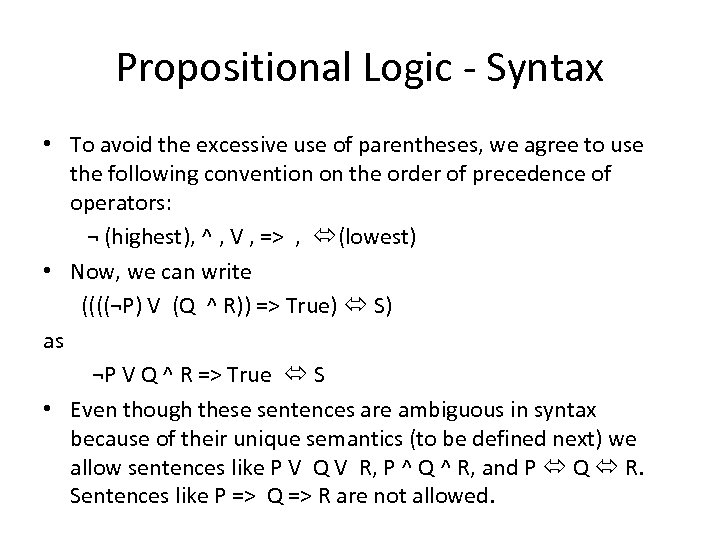Propositional Logic - Syntax • To avoid the excessive use of parentheses, we agree to use the following convention on the order of precedence of operators: ¬ (highest), ^ , V , => , (lowest) • Now, we can write ((((¬P) V (Q ^ R)) => True) S) as ¬P V Q ^ R => True S • Even though these sentences are ambiguous in syntax because of their unique semantics (to be defined next) we allow sentences like P V Q V R, P ^ Q ^ R, and P Q R. Sentences like P => Q => R are not allowed.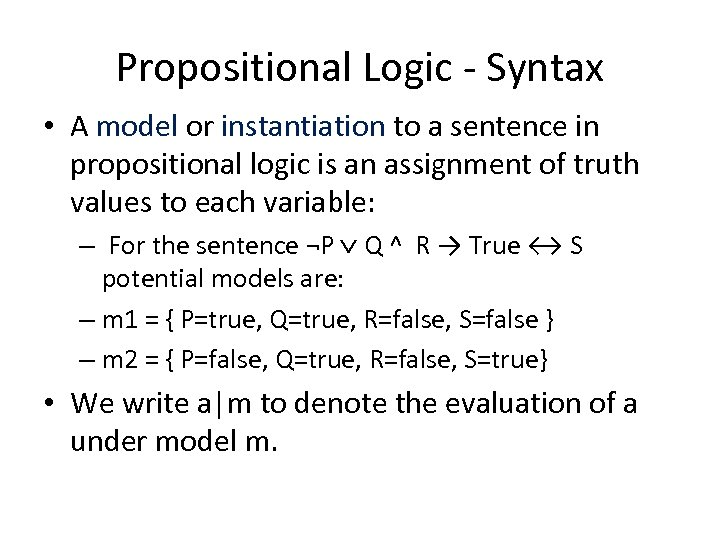Propositional Logic - Syntax • A model or instantiation to a sentence in propositional logic is an assignment of truth values to each variable: – For the sentence ¬P Q ^ R → True ↔ S potential models are: – m 1 = { P=true, Q=true, R=false, S=false } – m 2 = { P=false, Q=true, R=false, S=true} • We write a|m to denote the evaluation of a under model m.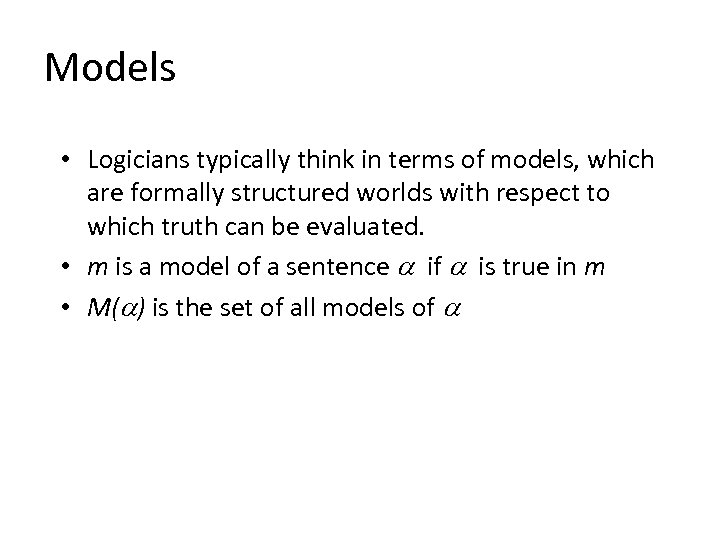Models • Logicians typically think in terms of models, which are formally structured worlds with respect to which truth can be evaluated. • m is a model of a sentence if is true in m • M( ) is the set of all models of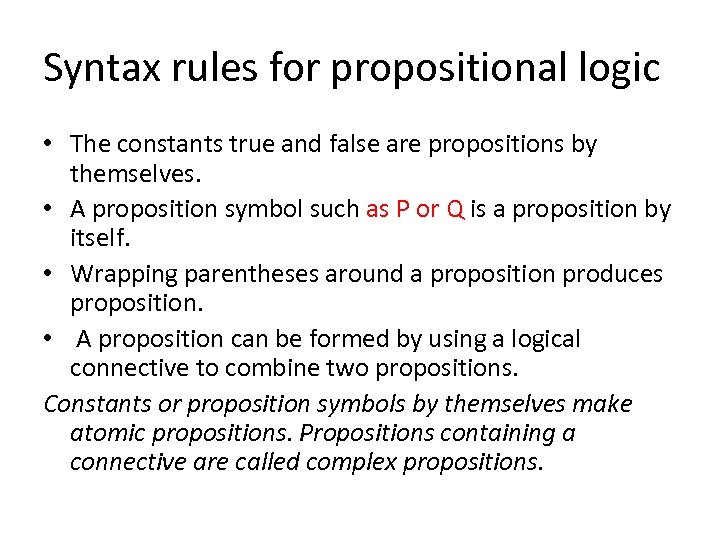Syntax rules for propositional logic • The constants true and false are propositions by themselves. • A proposition symbol such as P or Q is a proposition by itself. • Wrapping parentheses around a proposition produces proposition. • A proposition can be formed by using a logical connective to combine two propositions. Constants or proposition symbols by themselves make atomic propositions. Propositions containing a connective are called complex propositions.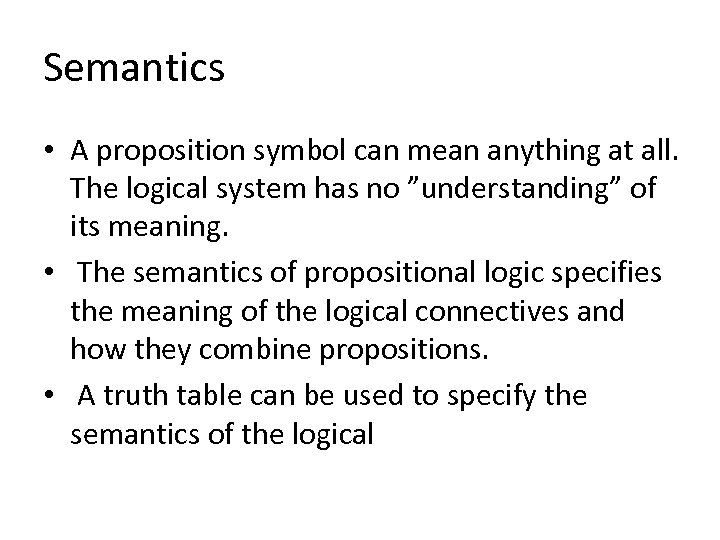Semantics • A proposition symbol can mean anything at all. The logical system has no ”understanding” of its meaning. • The semantics of propositional logic specifies the meaning of the logical connectives and how they combine propositions. • A truth table can be used to specify the semantics of the logical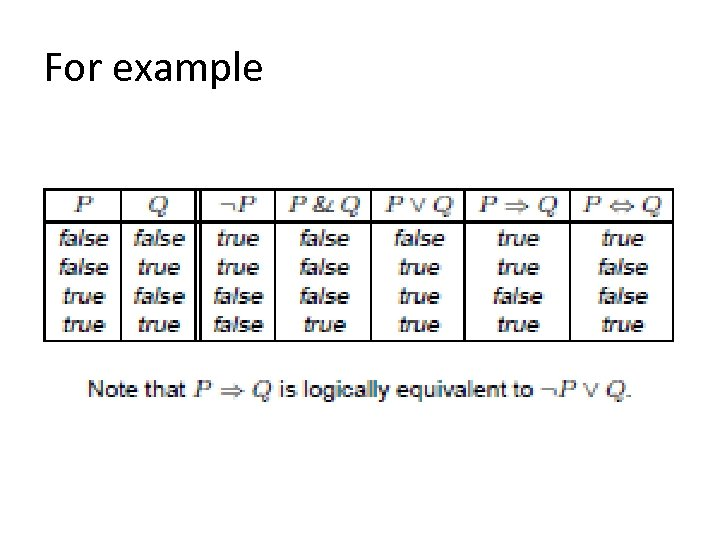For example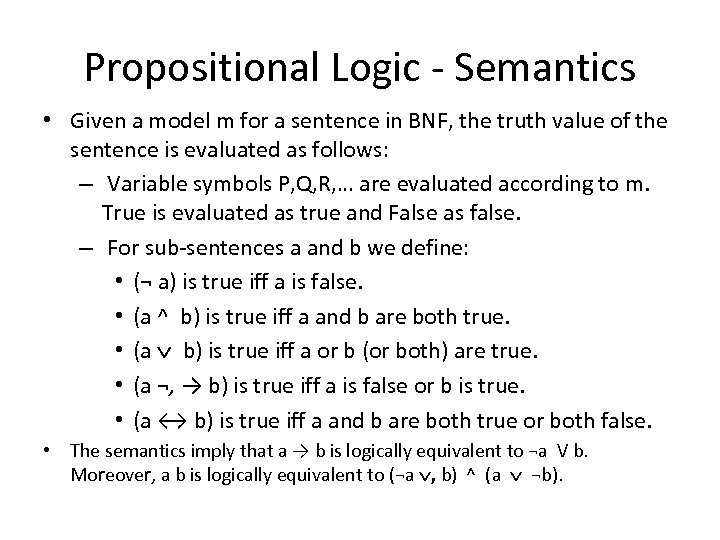Propositional Logic - Semantics • Given a model m for a sentence in BNF, the truth value of the sentence is evaluated as follows: – Variable symbols P, Q, R, … are evaluated according to m. True is evaluated as true and False as false. – For sub-sentences a and b we define: • (¬ a) is true iff a is false. • (a ^ b) is true iff a and b are both true. • (a b) is true iff a or b (or both) are true. • (a ¬, → b) is true iff a is false or b is true. • (a ↔ b) is true iff a and b are both true or both false. • The semantics imply that a → b is logically equivalent to ¬a V b. Moreover, a b is logically equivalent to (¬a , b) ^ (a ¬b).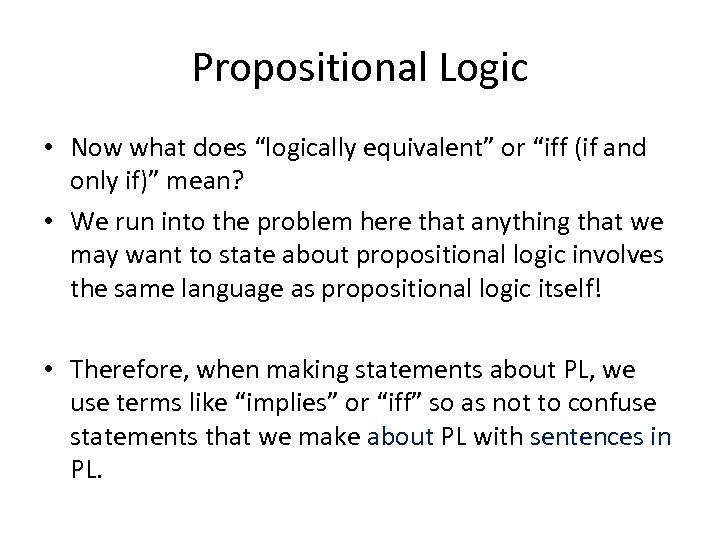Propositional Logic • Now what does “logically equivalent” or “iff (if and only if)” mean? • We run into the problem here that anything that we may want to state about propositional logic involves the same language as propositional logic itself! • Therefore, when making statements about PL, we use terms like “implies” or “iff” so as not to confuse statements that we make about PL with sentences in PL.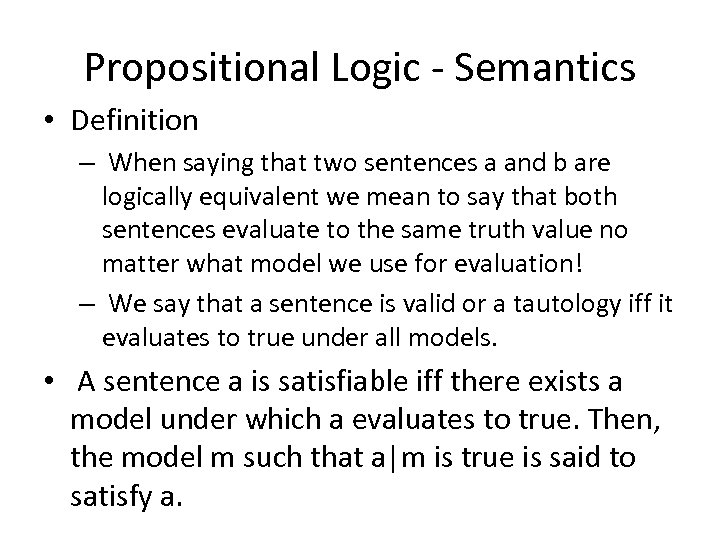Propositional Logic - Semantics • Definition – When saying that two sentences a and b are logically equivalent we mean to say that both sentences evaluate to the same truth value no matter what model we use for evaluation! – We say that a sentence is valid or a tautology iff it evaluates to true under all models. • A sentence a is satisfiable iff there exists a model under which a evaluates to true. Then, the model m such that a|m is true is said to satisfy a.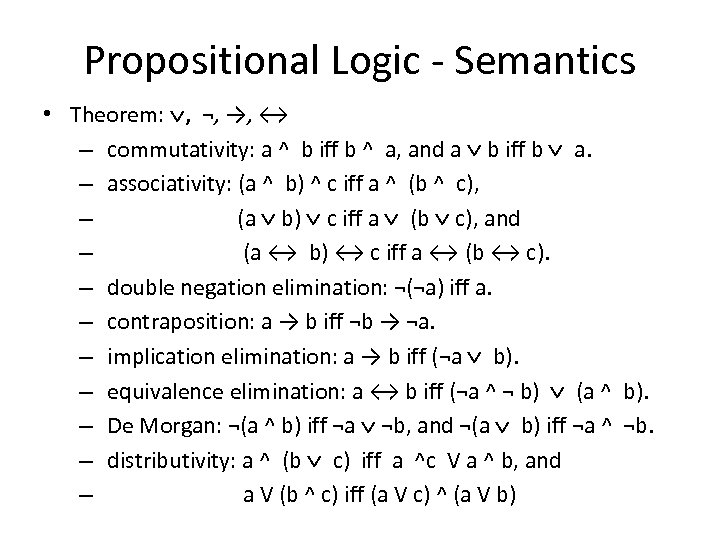Propositional Logic - Semantics • Theorem: , ¬, →, ↔ – commutativity: a ^ b iff b ^ a, and a b iff b a. – associativity: (a ^ b) ^ c iff a ^ (b ^ c), – (a b) c iff a (b c), and – (a ↔ b) ↔ c iff a ↔ (b ↔ c). – double negation elimination: ¬(¬a) iff a. – contraposition: a → b iff ¬b → ¬a. – implication elimination: a → b iff (¬a b). – equivalence elimination: a ↔ b iff (¬a ^ ¬ b) (a ^ b). – De Morgan: ¬(a ^ b) iff ¬a ¬b, and ¬(a b) iff ¬a ^ ¬b. – distributivity: a ^ (b c) iff a ^c V a ^ b, and – a V (b ^ c) iff (a V c) ^ (a V b)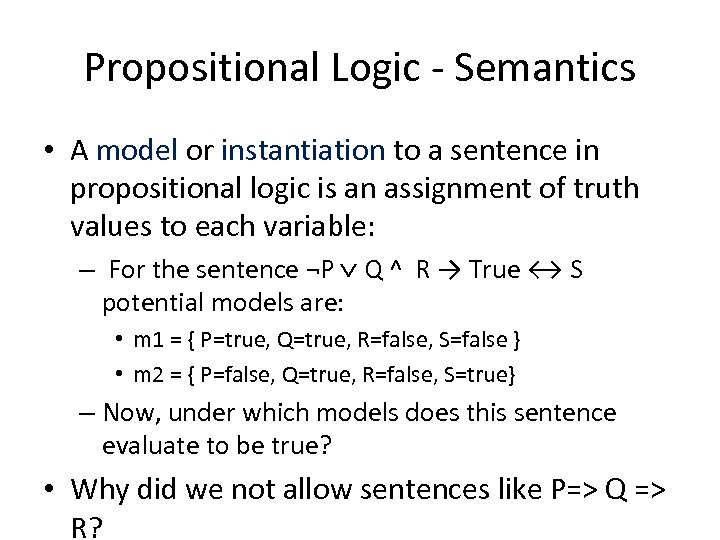Propositional Logic - Semantics • A model or instantiation to a sentence in propositional logic is an assignment of truth values to each variable: – For the sentence ¬P Q ^ R → True ↔ S potential models are: • m 1 = { P=true, Q=true, R=false, S=false } • m 2 = { P=false, Q=true, R=false, S=true} – Now, under which models does this sentence evaluate to be true? • Why did we not allow sentences like P=> Q => R?